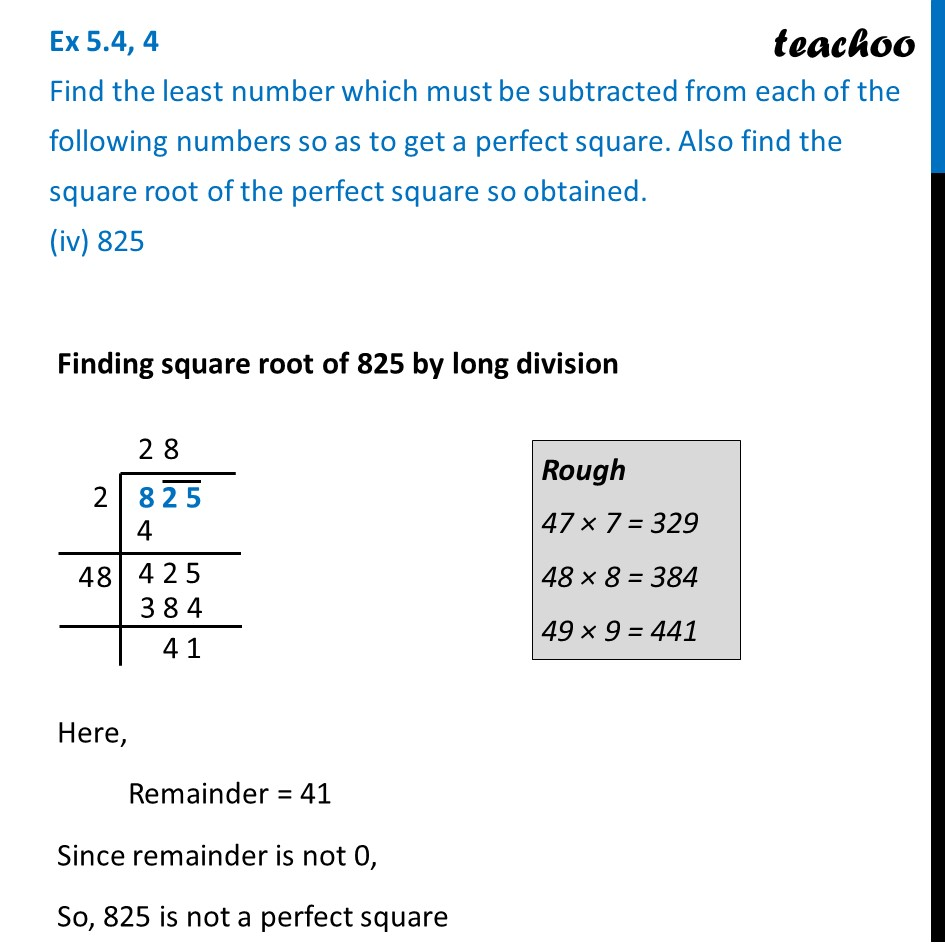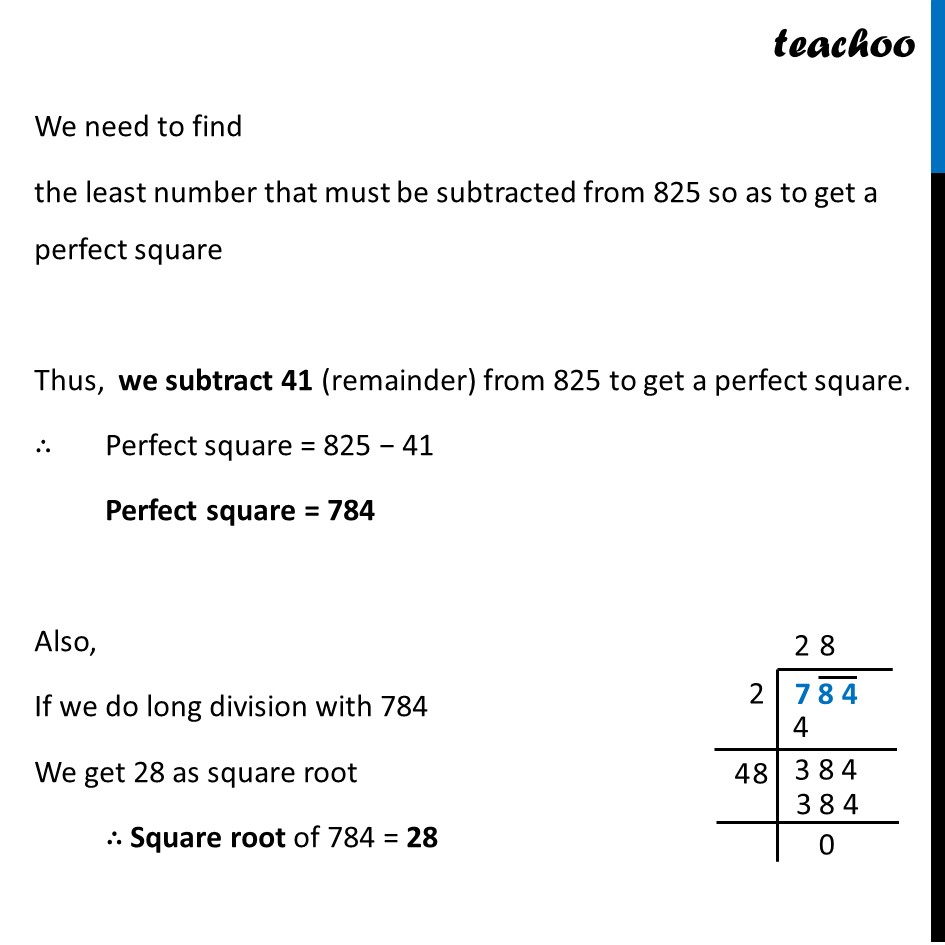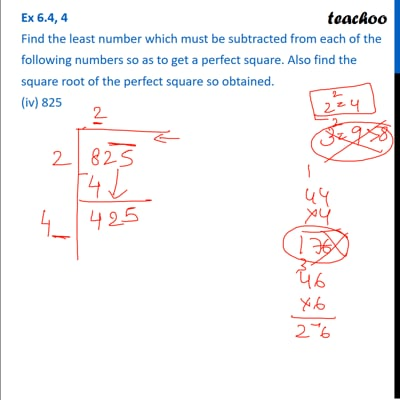Ex 5.4

Chapter 5 Class 8 Squares and Square Roots
Serial order wiseThis video is only available for Teachoo black users

Learn in your speed, with individual attention - Teachoo Maths 1-on-1 Class

### Transcript

Ex 5.4, 4 Find the least number which must be subtracted from each of the following numbers so as to get a perfect square. Also find the square root of the perfect square so obtained. (iv) 825 Finding square root of 825 by long division Here, Remainder = 41 Since remainder is not 0, So, 825 is not a perfect square We need to find the least number that must be subtracted from 825 so as to get a perfect square Thus, we subtract 41 (remainder) from 825 to get a perfect square. ∴ Perfect square = 825 − 41 Perfect square = 784 Also, If we do long division with 784 We get 28 as square root ∴ Square root of 784 = 28